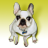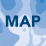# Need very urgent help with LS Factor(USLE)Calculation in ArcGis10 (get strange result

11048
10
08-12-2012 09:44 AMNew Contributor
I wanted to calculate the LS Factor of the USLE (Universal Soil Loss Equation) with the tool Raster Calculator in ArcGis 10.
First, I filled my DEM with the tool Fill. (DEM was calculated in UTM)
Second, I calculated the Flow Direction with the Tool Flow Direction.
Third, I calculated the Flow Accumulation with my Flow Direction as Inputfile. [ATTACH=CONFIG]16862[/ATTACH]
Fourth, I calculated the Slope in Degrees by the Spatial Analyst tool Surface and Slope. [ATTACH=CONFIG]16863[/ATTACH]
Fifthly, I used the Equation by Moore and Burch 1986: LS = (Flow Accumulation * Cell Size/22.13)^0.4 * (sin (slope/0.0896))^1.3
Sixthly: I typed in the raster calculator the equation:

(Power((("FlowAcc" * 92.46745509) / 22.13),0.4)) * (Power((Sin("Slope_Degree" / 0.0896)),1.3))

But my result look very very strange! There a lot of white areas! The Raster is not like cover areas.... It looks like that every calculated value is seperated... It looks like a dotted map....What did I wrong?? Did I forget something?? I can`t believe that this is right??!! [ATTACH=CONFIG]16864[/ATTACH]
Tags (4)
10 RepliesNew Contributor
Hello!

At first you have to investigate your map. Are these white areas NoData? Which values are in other layers where NoData values appear in LS layer. It seems that there is a problem with FlowAccumulation layer - there also seem to have NoData values.

I have used Wischmeier and Smith's LS factor formula and it works:

Pow(([FlowLength]/22.13), [m_factor] / 10)*(((Sin([Slope_degrees] / deg)*Sin([Slope_degrees] / deg))*65.41)+(Sin([Slope_degrees] / deg)*4.56)+0.065)

FlowLength substitues slope length, like FlowAccumulation
m factor is 0,2 for slopes less than 0.01% (slope_percent), 0,3 for slopes 0,01-0,035, 0,4 for slopes 0,035-0,05 and 0,5 for slopes over 0,05%. As the reclassify tool does not enable to give new values like 0,2 then just integer values (like 2) have to assigned and these vales are divided by 10 in formula (m_factor / 10)
deg - constant, 57,296

I hope this helped,
Anneby Anonymous User
Not applicable
Original User: Julia.Hauczinger

Thank you Anne Kull!

Yes I investigeted my DEM, Fill, Flowdir and Accumulation... there are no missing data, where the missing data in my LS Layer are...

I would like to try this equation, but I have there a question:
"FlowLength substitues slope length, like FlowAccumulation
m factor is 0,2 for slopes less than 0.01% (slope_percent), 0,3 for slopes 0,01-0,035, 0,4 for slopes 0,035-0,05 and 0,5 for slopes over 0,05%. As the reclassify tool does not enable to give new values like 0,2 then just integer values (like 2) have to assigned and these vales are divided by 10 in formula (m_factor / 10)
deg - constant, 57,296"

Did you calculate this in Raster Calculator? I have absolutly no idea how do this part with the different values for m dependening on the different slope! How can I say, that it has to take for m the value 0,2 for the slope 0,01%, and take the value 0,3 for 0,01 to 0,035,... ???? (Sorry I am just a beginner in GIS and I try to learn 🙂 )

Or have I to calculate it somehow else?New Contributor
At first I prepared the m factor layer. To do this I calculated the slope in percent and then classified (Reclassify tool) the slope_percent layer to have m factor classes:
0-0,01% = 2
0,01-0,035% = 3
0,035-0,05% = 4
above 0,05% = 5

And then I calculated with Raster Calculator the LS factor using formula I wrote. This formula is written by ArcGIS 9.3. In ArcGIS 9.3 the syntax looks like little bit different - there are  quatation marks "" around layer names instead of square brackes [].

Anneby Anonymous User
Not applicable
Original User: Julia.Hauczinger

Thank you very very much for your help and your good explanation!!!! 🙂by Anonymous User
Not applicable
Original User: amar.gautam

hi Julia Hauczinger,
i am also working on USLE equation. I follow the same steps as given in this post but didn't get the right solution.
If you get another solution please tell me.

with regard
AmaRNew Contributor
why do we need to divide by the deg - which is the constant of 57,296, This is the part I am lost at.New Contributor

In the Raster Calculator: sin(angle)

The "angle" must be in radians; therefore, the correction to your formula would be:

(Power((("FlowAcc" * 92.46745509) / 22.13),0.4)) * (Power((Sin("Slope_Degree" * 0.01745 / 0.0896)),1.3))

Please let me know if it works.

Regards.New Contributor

Hi Julia,

please look the Gisus-M. You ca see it HERE​.New Contributor

Hi JoanitoOliveira,

I tried and installed the GISus_M esri add-in  in Arcgis 10.2, however, it's not functional. Just it's only installed and also showing in Arc tool list, but not highlighting any the options in the model.  Kindly suggest me right procedure for installation in order to work on the model.

Thanks